1602903600

# Deformable Convolutions Demystified

## Prerequisites:

The reader of the post must have a basic understanding of Convolutional Neural Networks. If you are unfamiliar with the topic you can refer to this**link **and if you want to know more about the convolutional operation which is actually derived from basic image processing, you can read this blogpost as well.

## Introduction

Convolutional Neural Networks or CNN’s, in short, is one of the main causes of the revival of artificial intelligence research after a very long AI winter. The applications based on them were the first ones which showcased the power of artificial intelligence or deep learning to be precise and revived the faith in the field which was lost after Marvin Minsky pointed out that Perceptron just worked on linearly separable data and failed to work on the simplest non-linear functions such as XOR.

Convolutional Neural Networks are very popular in the domain of Computer Vision and almost all state of the art applications such as google images, self-driving cars etc are based on them. In very high-level, they are a kind of neural network which focus on local spatial information and use weight sharing to extract features in a hierarchical manner which are finally aggregated in some task-specific manner to give the task-specific output.

Though CNN’s are excellent for visual-recognition tasks but are very limited when it comes to modelling geometric variations or geometric transformations in object scale, pose, viewpoint and part deformation.

Geometric Transformations are basic transformations which transform the positions and orientation of an image to another position and orientation.

Some basic geometric transformations are scaling, rotation, translating etc.

Convolutional Neural Networks lack an internal mechanism to model geometric variations and can only model them using data-augmentations which are fixed and limited by the user’s knowledge and hence the CNN cannot learn geometric transformations unknown to the user.

To overcome this problem and increase the capabilities of CNN, Deformable Convolutionswere introduced by Microsoft Research Asia. In their work, they introduced a simpleefficient and end-to-end mechanism which makes the CNN capable of learning various geometric transformations according to the given data.

#neural-networks #machine-learning #computer-vision #deep-learning

## Buddha Community1597277640

## Deriving convolution from first principles

##### Basics Of Deep Learning

TL;DR:_ Have you even wondered what is so special about convolution? In this post, I derive the convolution from first principles and show that it naturally emerges from translational symmetry._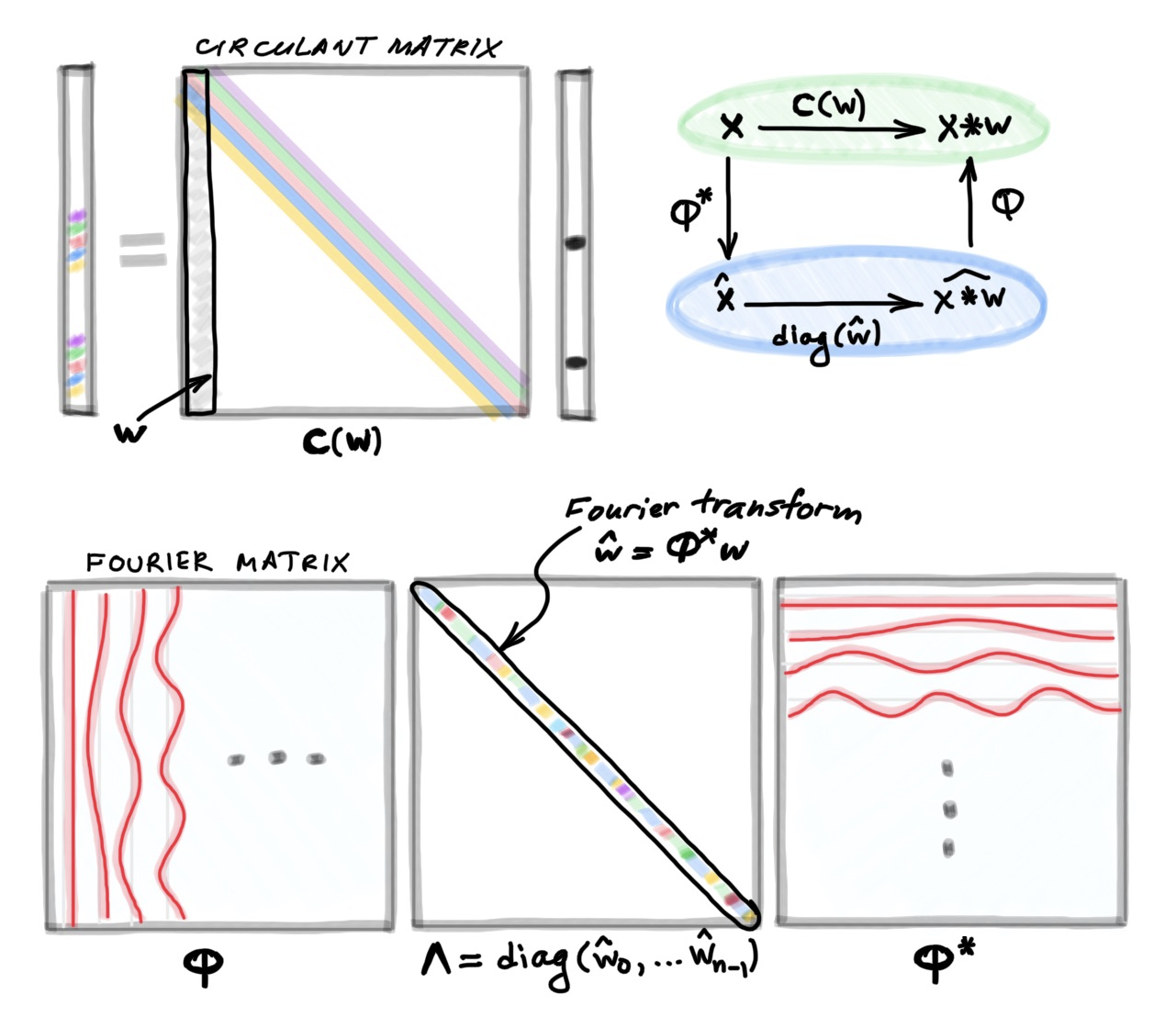La connoissance de certains principes supplée facilement à la connoissance de certains faits. (Claude Adrien Helvétius)

During my undergraduate studies, which I did in Electrical Engineering at the Technion in Israel, I was always appalled that such an important concept as convolution  just landed out of nowhere. This seemingly arbitrary definition disturbed the otherwise beautiful picture of the signal processing world like a grain of sand in one’s eye. How nice would it be to have the convolution emerge from first principles rather than have it postulated! As I will show in this post, such first principles are the notion of translational invariance or symmetry.

Let me start with the formula taught in basic signal processing courses defining the discrete convolution  of two n-dimensional vectors x and w:Here, for convenience, I assume that all the indices run from zero to _n_−1 and are modulo n; it is convenient to think of vectors as defined on a circle. Writing the above formula as a matrix-vector multiplication leads to a very special matrix that is called circulant: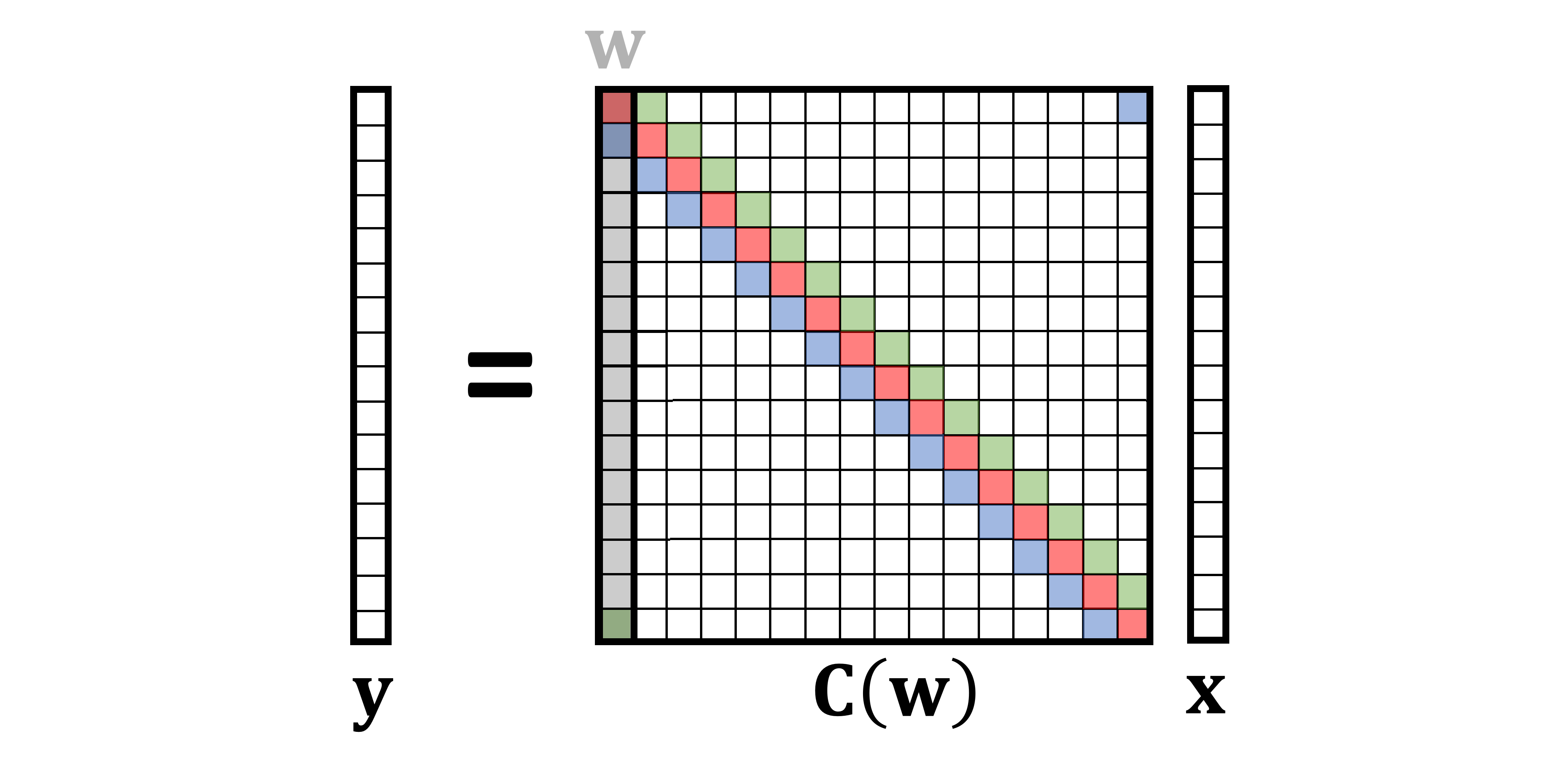A circulant matrix has multi-diagonal structure, with elements on each diagonal having the same value. It can be formed by stacking together shifted (modulo n) versions of a vector ; for this reason, I use the notation C(w) referring to a circulant matrix formed by the vector w. Since any convolution xwcan beequivalently represented as a multiplication by the circulant matrix C(w)x, I will use the two terms interchangeably.

#ai & machine learning #convolution #convolutional newral net #deep learning #deep learning1596633180

## Deriving convolution from first principles

TL;DR:_ Have you even wondered what is so special about convolution? In this post, I derive the convolution from first principles and show that it naturally emerges from translational symmetry._La connoissance de certains principes supplée facilement à la connoissance de certains faits. (Claude Adrien Helvétius)

D

uring my undergraduate studies, which I did in Electrical Engineering at the Technion in Israel, I was always appalled that such an important concept as convolution  just landed out of nowhere. This seemingly arbitrary definition disturbed the otherwise beautiful picture of the signal processing world like a grain of sand in one’s eye. How nice would it be to have the convolution emerge from first principles rather than have it postulated! As I will show in this post, such first principles are the notion of translational invariance or symmetry.

Let me start with the formula taught in basic signal processing courses defining the discrete convolution  of two n-dimensional vectors x and w:Here, for convenience, I assume that all the indices run from zero to _n_−1 and are modulo n; it is convenient to think of vectors as defined on a circle. Writing the above formula as a matrix-vector multiplication leads to a very special matrix that is called circulant: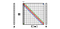A circulant matrix has multi-diagonal structure, with elements on each diagonal having the same value. It can be formed by stacking together shifted (modulo n) versions of a vector ; for this reason, I use the notation C(w) referring to a circulant matrix formed by the vector w. Since any convolution xwcan beequivalently represented as a multiplication by the circulant matrix C(w)x, I will use the two terms interchangeably.

One of the first things we are taught in linear algebra is that matrix multiplication is non-commutative, i.e.,in general, ABBA. However, circulant matrices are very special exception:

Circulant matrices commute,

or in other words, C(w)C(u)=C(u)C(w). This is true for any circulant matrix, or any choice of u and w. Equivalently, we can say that the convolution is a commutative operation, xw=wx.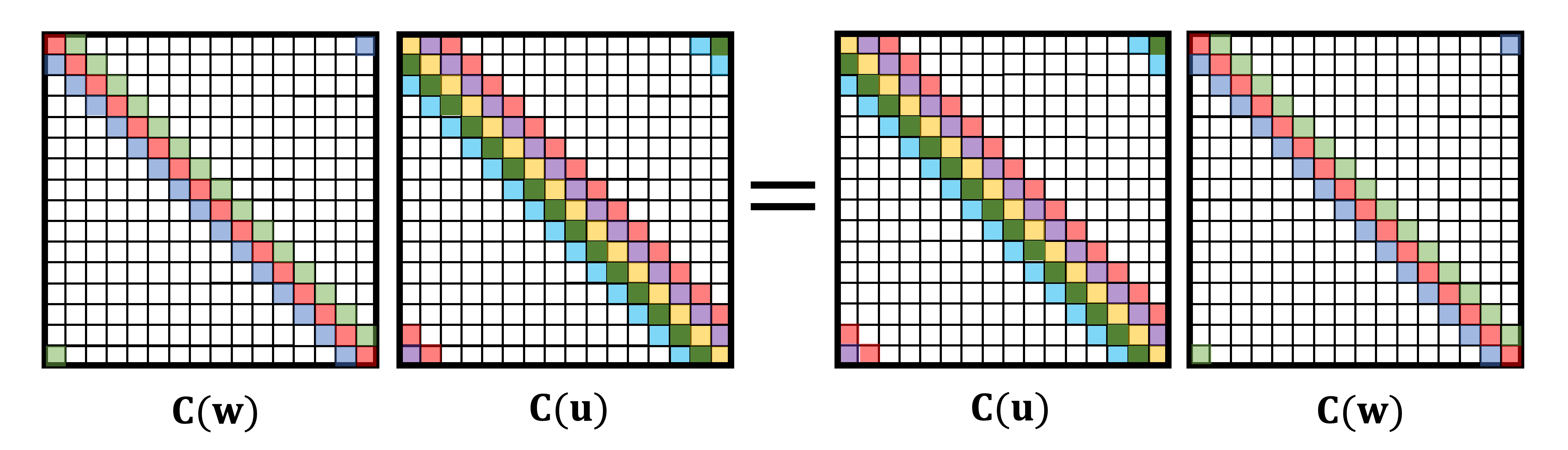A particular choice of w=[0,1,0…,0] yields a special circulant matrix that shifts vectors to the right by one position. This matrix is called the (right) _shift operator _ and denoted by S. The transpose of the right shift operator is the left shift operator. Obviously, shifting left and then right (or vice versa) does not do anything, which means S is an orthogonal matrix: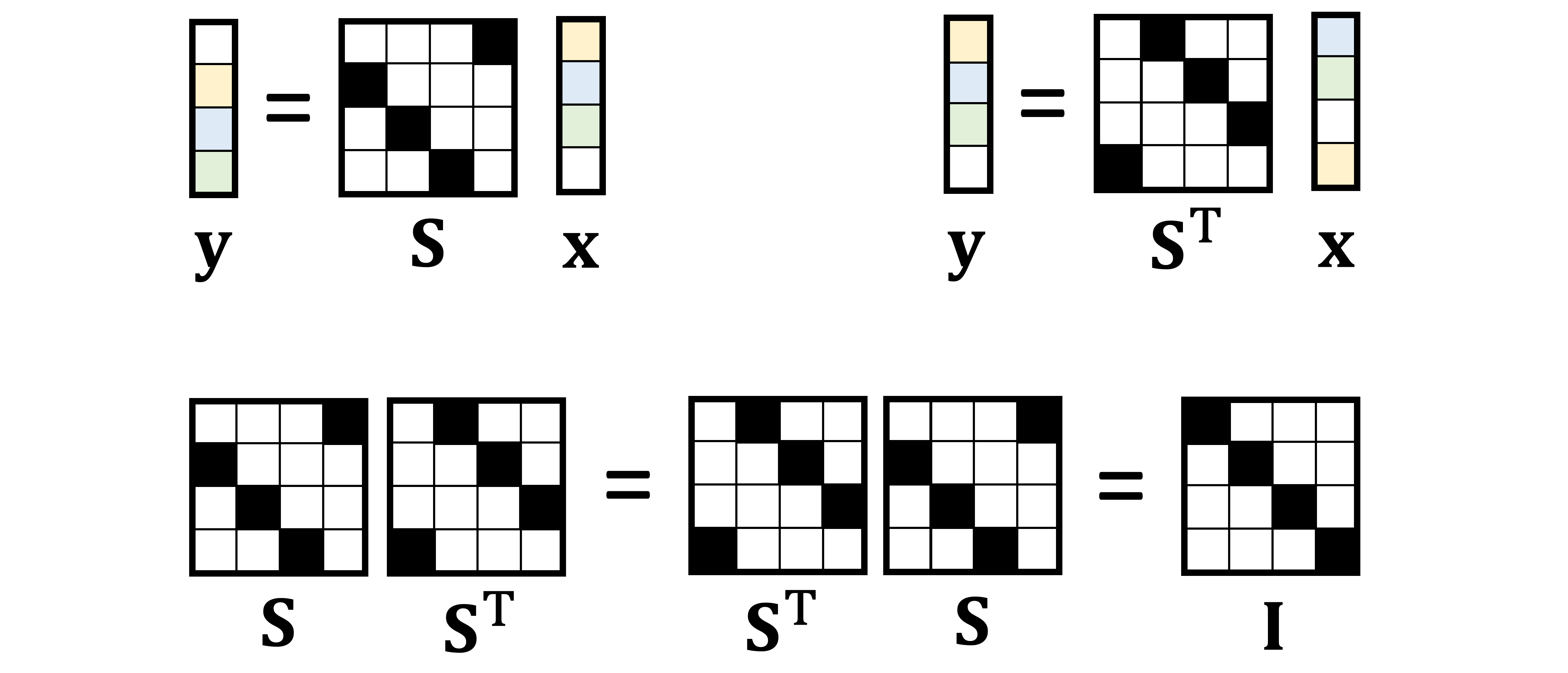Circulant matrices can be characterised by their commutativity property. It appears to be sufficient to show only commutativity with shift (Lemma 3.1 in ):

A matrix is circulant if and only if it commutes with shift.

The first direction of this “if and only if” statement leads to a very important property called translation or _shift equivariance _: the convolution’s commutativity with shift implies that it does not matter whether we first shift a vector and then convolve it, or first convolve and then shift — the result will be the same.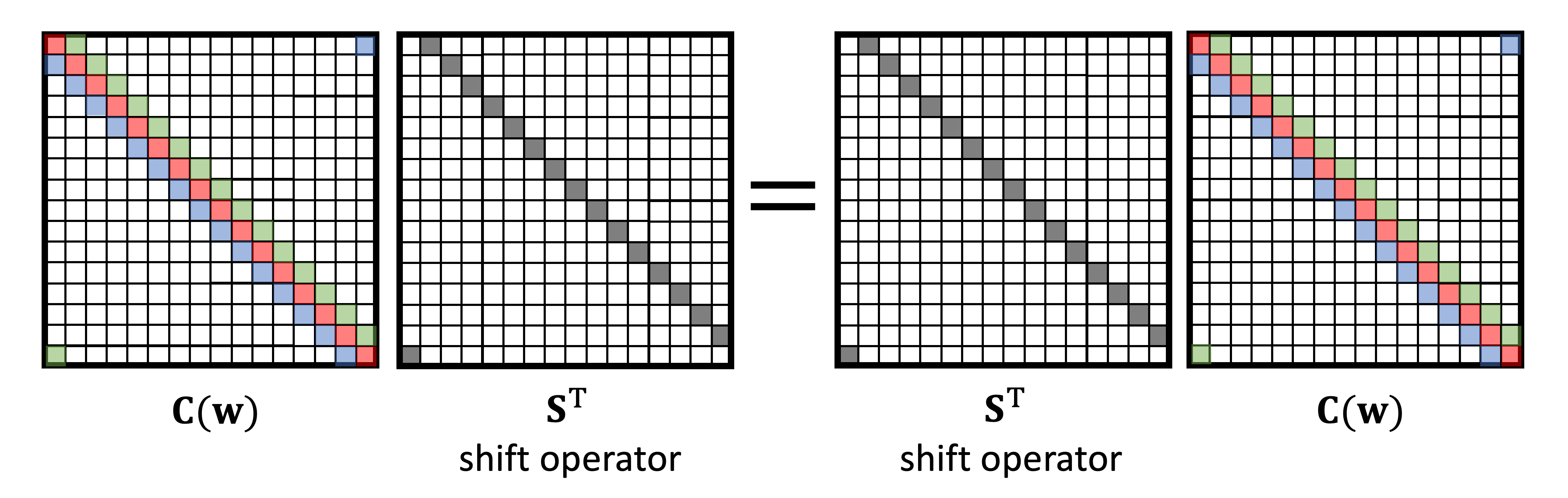The second direction allows us to define convolution as the shift-equivariant linear operation: in order to commute with shift, a matrix must have the circulant structure. This is exactly what we aspired to from the beginning, to have the convolution emerge from the first principles of translational symmetry . Instead of being given a formula of the convolution and proving its shift equivariance property, as it is typically done in signal processing books, we can start from the requirement of shift equivariance and arrive at the formula of the convolution as the only possible linear operation satisfying it.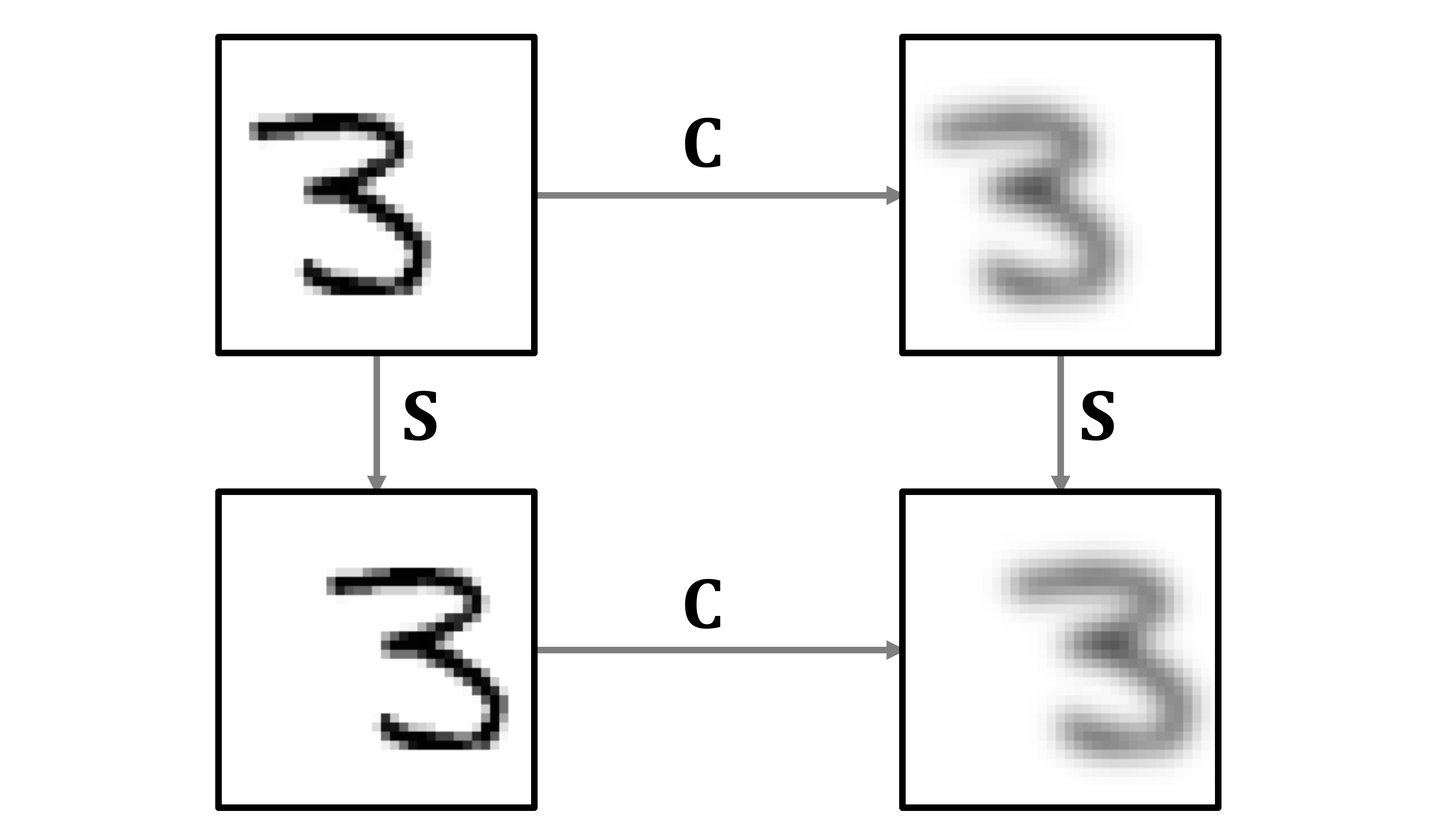Illustration of shift equivariance as the interchangeability of shift and blur operations.

A nother important fact taught in signal processing courses is the connection between the convolution and the Fourier transform . Here as well, the Fourier transform lands out of the blue, and then one is shown that it diagonalises the convolution operation, allowing to perform convolution of two vectors in the frequency domain as element-wise product of their Fourier transforms. Nobody ever explains where these sines and cosines come from and what is so special about them.

#deep-learning #convolutional-neural-net #data-science #machine-learning #convolution #deep learning1596826500

## Convolutional Neural Networks-An Intuitive approach

A simple yet comprehensive approach to the concepts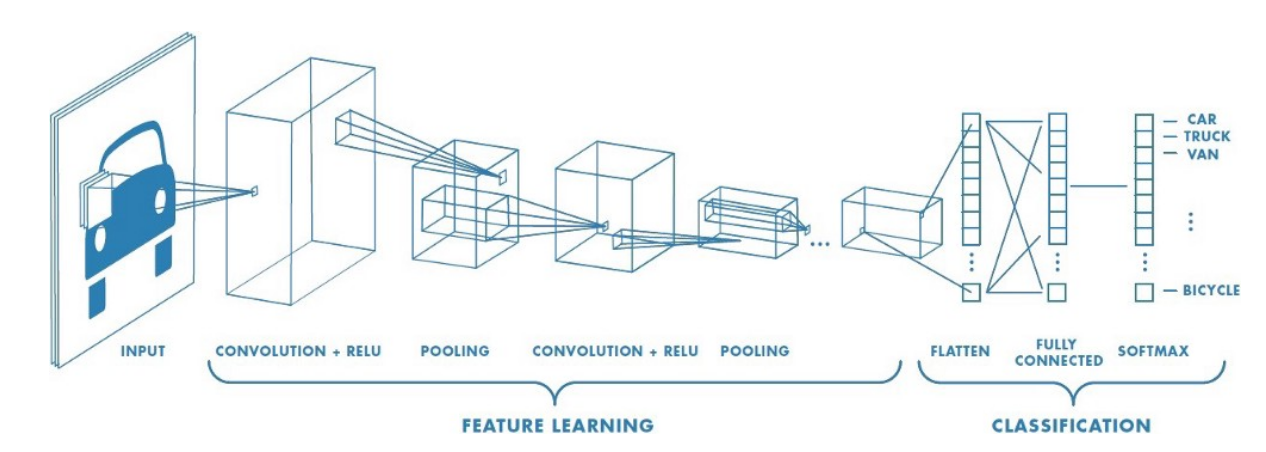Convolutional Neural Networks

Artificial intelligence has seen a tremendous growth over the last few years, The gap between machines and humans is slowly but steadily decreasing. One important difference between humans and machines is (or rather was!) with regards to human’s perception of images and sound.How do we train a machine to recognize images and sound as we do?

At this point we can ask ourselves a few questions!!!

How would the machines perceive images and sound ?

How would the machines be able to differentiate between different images for example say between a cat and a dog?

Can machines identify and differentiate between different human beings for example lets say differentiate a male from a female or identify Leonardo Di Caprio or Brad Pitt by just feeding their images to it?

Let’s attempt to find out!!!

The Colour coding system:

Lets get a basic idea of what the colour coding system for machines is

RGB decimal system: It is denoted as rgb(255, 0, 0). It consists of three channels representing RED , BLUE and GREEN respectively . RGB defines how much red, green or blue value you’d like to have displayed in a decimal value somewhere between 0, which is no representation of the color, and 255, the highest possible concentration of the color. So, in the example rgb(255, 0, 0), we’d get a very bright red. If we wanted all green, our RGB would be rgb(0, 255, 0). For a simple blue, it would be rgb(0, 0, 255).As we know all colours can be obtained as a combination of Red , Green and Blue , we can obtain the coding for any colour we want.

Gray scale: Gray scale consists of just 1 channel (0 to 255)with 0 representing black and 255 representing white. The colors in between represent the different shades of Gray.

Computers ‘see’ in a different way than we do. Their world consists of only numbers.

Every image can be represented as 2-dimensional arrays of numbers, known as pixels.

But the fact that they perceive images in a different way, doesn’t mean we can’t train them to recognize patterns, like we do. We just have to think of what an image is in a different way.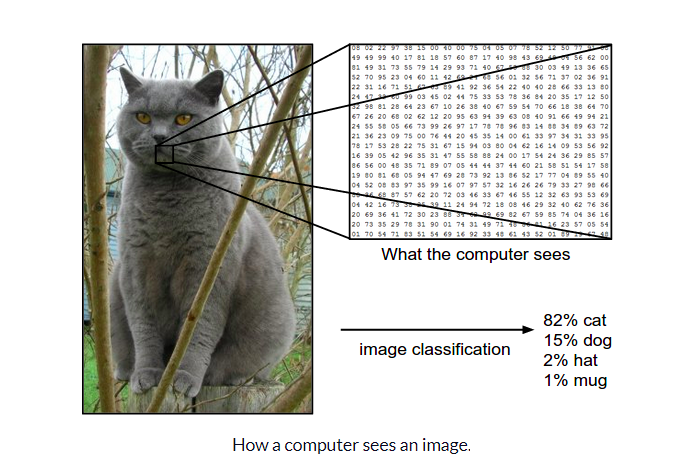Now that we have a basic idea of how images can be represented , let us try and understand The architecture of a CNN

CNN architecture

Convolutional Neural Networks have a different architecture than regular Neural Networks. Regular Neural Networks transform an input by putting it through a series of hidden layers. Every layer is made up of a set of neurons, where each layer is fully connected to all neurons in the layer before. Finally, there is a last fully-connected layer — the output layer — that represent the predictions.

Convolutional Neural Networks are a bit different. First of all, the layers are organised in 3 dimensions: width, height and depth. Further, the neurons in one layer do not connect to all the neurons in the next layer but only to a small region of it. Lastly, the final output will be reduced to a single vector of probability scores, organized along the depth dimension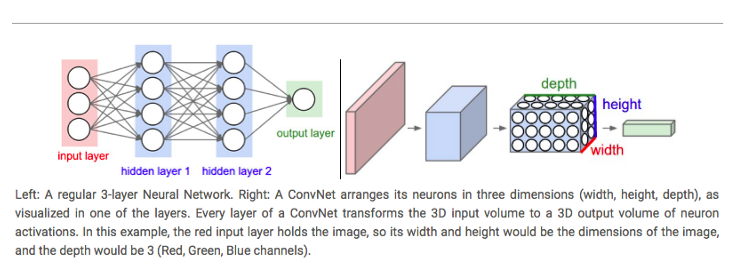A typical CNN architecture

As can be seen above CNNs have two components:

• The Hidden layers/Feature extraction part

In this part, the network will perform a series of **convolutions **and pooling operations during which the features are detected. If you had a picture of a tiger , this is the part where the network would recognize the stripes , 4 legs , 2 eyes , one nose , distinctive orange colour etc.

• The Classification part

Here, the fully connected layers will serve as a classifier on top of these extracted features. They will assign a** probability** for the object on the image being what the algorithm predicts it is.

Before we proceed any further we need to understand what is “convolution”, we will come back to the architecture later:

What do we mean by the “convolution” in Convolutional Neural Networks?

Let us decode!!!

#convolutional-neural-net #convolution #computer-vision #neural networks1615979880

## Convolutional AutoEncoders (CAE) with Tensorflow

Autoencoders has been in the deep learning literature for a long time now, most popular for data compression tasks. With their easy structure and not so complicated underlying mathematics, they became one of the first choices when it comes to dimensionality reduction in simple data. However, using basic fully connected layers fail to capture the patterns in pixel-data since they do not hold the neighboring information. For a good capturing of the image data in latent variables, convolutional layers are usually used in autoencoders.
Introduction
Autoencoders are unsupervised neural network models that summarize the general properties of data in fewer parameters while learning how to reconstruct it after compression. In order to extract the textural features of images, convolutional neural networks provide a better architecture. Moreover, CAEs can be stacked in such a way that each CAE takes the latent representation of the previous CAE for higher-level representations. Nevertheless, in this article, a simple CAE will be implemented having 3 convolutional layers and 3 subsampling layers in between.
The tricky part of CAEs is at the decoder side of the model. During encoding, the image sizes get shrunk by subsampling with either average pooling or max-pooling. Both operations result in information loss which is hard to re-obtain while decoding.

#convolutional-network #tensorflow #deep-learning #artificial-intelligence #convolutional-autoencoder1595436720

## Semantic Image Segmentation using Fully Convolutional Networks

Humans have the innate ability to identify the objects that they see in the world around them. The visual cortex present in our brain can distinguish between a cat and a dog effortlessly in almost no time. This is true not only with cats and dogs but with almost all the objects that we see. But a computer is not as smart as a human brain to be able to this on its own. Over the past few decades, Deep Learning researchers have tried to bridge this gap between human brain and computer through a special type of artificial neural networks called Convolutional Neural Networks(CNNs).

# What is a Convolutional Neural Network?

After a lot of research to study mammalian brains, researchers found that specific parts of the brain get activated to specific type of stimulus. For example, some parts in the visual cortex get activated when we see vertical edges, some when we see horizontal edges, and some others when we see specific shapes, colors, faces, etc. ML researchers imagined each of these parts as a layer of neural network and considered the idea that a large network of such layers could mimic the human brain.

This intuition gave rise to the advent of CNN, which is a type of neural network whose building blocks are convolutional layers. A convolution layer is nothing but a set of weight matrices called kernels or filters which are used for convolution operation on a feature matrix such as an image.Convolution:

2D convolution is a fairly simple operation, you start with a kernel and ‘stride’ (slide) it over the 2D input data, performing an element-wise multiplication with the part of the input it is currently on, and then summing up the results into a single output cell. The kernel repeats this process for every location it slides over, converting a 2D matrix of features into another 2D matrix of features.

The step size by which the kernel slides on the input feature matrix is called stride. In the below animation, the input matrix has been added with an extra stripe of zeros from all four sides to ensure that the output matrix is of the same size as the input matrix. This is called (zero)padding.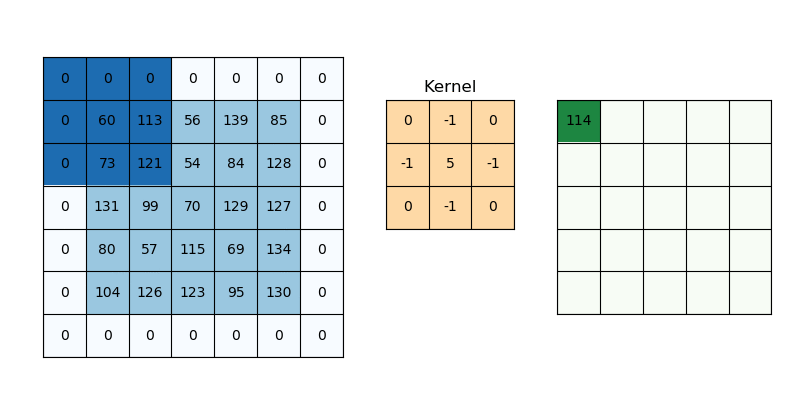2D Convolution: kernel size=3x3, padding=1 or ‘same’, stride=1

# Semantic Image Segmentation

Image segmentation is the task of partitioning a digital image into multiple segments (sets of pixels) based on some characteristics. The objective is to simplify or change the image into a representation that is more meaningful and easier to analyze.

Semantic Segmentation refers to assigning a class label to each pixel in the given image. See the below example.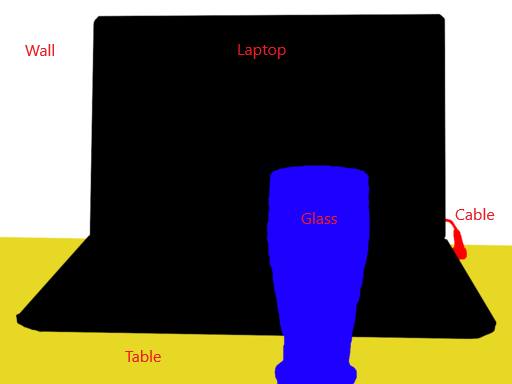Note that segmentation is different from classification. In classification, complete image is assigned a class label whereas in segmentation, each pixel in an image is classified into one of the classes.

Having a fair idea about convolutional networks and semantic image segmentation, let’s jump into the problem we need to solve.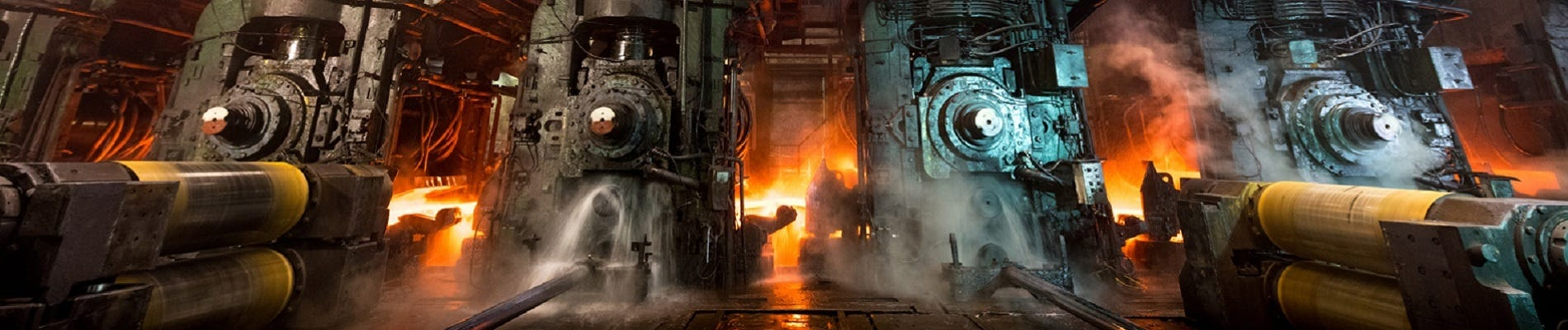Severstal is among the top 50 producers of steel in the world and Russia’s biggest player in efficient steel mining and production. One of the key products of Severstal is steel sheets. The production process of flat sheet steel is delicate. From heating and rolling, to drying and cutting, several machines touch flat steel by the time it’s ready to ship. To ensure quality in the production of steel sheets, today, Severstal uses images from high-frequency cameras to power a defect detection algorithm.

## Through this competition, Severstal expects the AI community to improve the algorithm by localizing and classifying surface defects on a steel sheet.Business objectives and constraints

1. A defective sheet must be predicted as defective since there would be serious concerns about quality if we misclassify a defective sheet as non-defective. i.e. high recall value for each of the classes is needed.We need not give the results for a given image in the blink of an eye. (No strict latency concerns)

# 2. Machine Learning Problem

2.1. Mapping the business problem to an ML problemOur task is to

1. Detect/localize the defects in a steel sheet using image segmentation andClassify the detected defects into one or more classes from [1, 2, 3, 4]

To put it together, it is a semantic image segmentation problem.2.2. Performance metricThe evaluation metric used is the mean Dice coefficient. The Dice coefficient can be used to compare the pixel-wise agreement between a predicted segmentation and its corresponding ground truth. The formula is given by:where X is the predicted set of pixels and Y is the ground truth.

Read more about Dice Coefficient here.2.3. Data OverviewWe have been given a zip folder of size 2GB which contains the following:

• `**train_images**` —a folder containing 12,568 training images (.jpg files)`**test_images**` — a folder containing 5506 test images (.jpg files). We need to detect and localize defects in these images`**train.csv**` — training annotations which provide segments for defects belonging to ClassId = [1, 2, 3, 4]`**sample_submission.csv**`— a sample submission file in the correct format, with each ImageId repeated 4 times, one for each of the 4 defect classes.

More details about data have been discussed in the next section.

# 3. Exploratory Data Analysis

The first step in solving any machine learning problem should be a thorough study of the raw data. This gives a fair idea about what our approaches to solving the problem should be. Very often, it also helps us find some latent aspects of the data which might be useful to our models.

## Let’s analyze the data and try to draw some meaningful conclusions.3.1. Loading train.csv file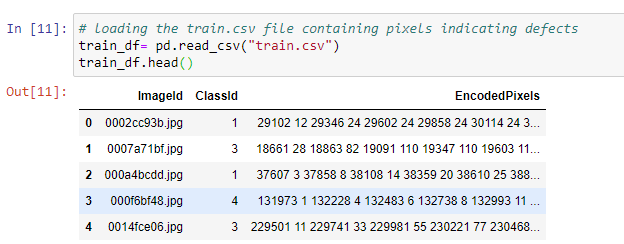**_train.csv _**tells which type of defect is present at what pixel location in an image. It contains the following columns:

• `**ImageId**`: image file name with .jpg extension`**ClassId**`: type/class of the defect, one of [1, 2, 3, 4]`**EncodedPixels**`: represents the range of defective pixels in an image in the form of run-length encoded pixels(pixel number where defect starts pixel length of the defect).
• e.g. ‘29102 12’ implies the defect is starting at pixel 29102 and running a total of 12 pixels, i.e. pixels 29102, 29103,………, 29113 are defective. The pixels are numbered from top to bottom, then left to right: 1 corresponds to pixel (1,1), 2 corresponds to (2,1), and so on.

#convolutional-network #semantic-segmentation #computer-vision #dilated-convolution #deep-learning #deep learning